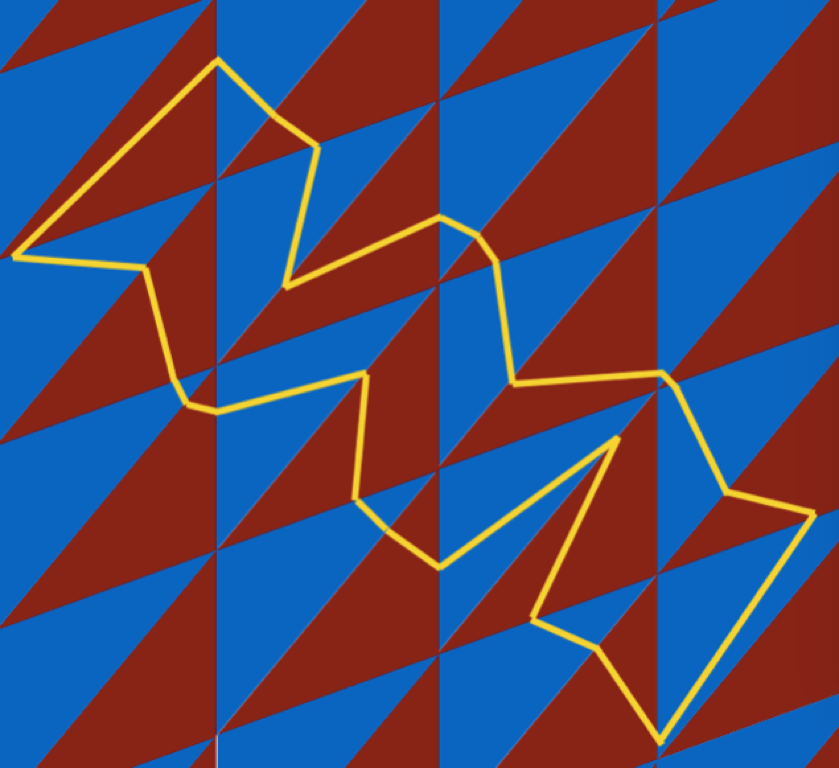# Complex Analysis and Dynamics Seminar

## Spring 2017 Schedule

#### Feb 10: Tamara Kucherenko (City College of CUNY) Ground States at the Boundary of Rotation Sets

Ground states are accumulation points of equilibrium states when the temperature goes to zero. They play a fundamental role in statistical physics. We consider rotation sets associated with a continuous dynamical system on a compact metric space and a multi-dimensional continuous potential. We study the question for which boundary vectors of the rotation set one can realize an entropy maximizing measure as a ground state associated with a certain linear combination of the potential. We show that at an exposed point there always exists a ground state that maximizes entropy in its rotation class. We also construct examples of rotation sets (in any dimension ) that have exposed boundary points without a ground state in its rotation class. Finally, we consider non-exposed points and show that the following three phenomena exist: a) boundary points without an associated ground state; b) boundary points with a unique ground state that is not ergodic; c) the set of rotation vectors of ground states of a certain linear combination of the potential is a non-trivial line segment.

#### Feb 17: The seminar will feature two talks: 2:00-2:50 Liviana Palmisano (University of Bristol) Foliations by Rigidity Classes

We prove that circle maps with a flat interval and degenerate geometry are an example of a dynamical system for which the topological classes do not coincide with the rigidity classes. Contrary to all well-known examples in one-dimensional dynamics (such as circle diffeomorphisms, unimodal interval maps at the boundary of chaos, critical circle maps), we show that the class of functions with Fibonacci rotation numbers is a $C^1$ manifold which is foliated with finite codimension rigidity classes. This is joint work with M. Martens.

#### 3:00-3:50 Alexandre Paiva Barreto (Federal University of Sao Carlos) Deformations of Hyperbolic Cone Structures

Unlike hyperbolic structures, which are rigid after Mostow Theorem, the hyperbolic cone structures can be deformed. The difficulty to understand these deformations lies in the possibility of degenerating the structure which occurs when the singular link intersects itself over the deformation. In this cases, the Hausdorff-Gromov limit of the deformation is just an Alexandrov space (of curvature bounded from below by $-1$) which may have dimension strictly smaller than $3$. In this talk we are interested in studying these deformations under the condition that the lengths of the singular link remain uniformly bounded over the deformation. This assumption avoids the undesirable case where the singularity becomes dense in the limiting Alexandrov space. The main theorem presented here will be used to bring some information to the following question proposed by W. Tursthon in 80's: Let $M$ be closed and orientable hyperbolic $3$-manifold and let $\Sigma$ be a simple closed geodesic in $M$. Can the hyperbolic structure of $M$ be deformed to the complete hyperbolic structure on $M-\Sigma$ through a path $M_{\alpha}$ of hyperbolic cone structures on $(M,\Sigma)$ with cone angles $\alpha \in [0,2\pi]$?

#### March 17: Ser-Wei Fu (Temple University) The Geometry of Quadratic Differentials on Surfaces

Quadratic differentials on a Riemann surface played a central role in Teichmuller theory. The interplay between complex structure, hyperbolic structure, and singular Euclidean geometry gave us plenty of insight in the study of the moduli space of Riemann surfaces. Instead of the geometry induced by a quadratic differential, I will talk about the geometry of the space of quadratic differentials. In particular, I will discuss a construction that can be used to generate paths between any two quadratic differentials. As a result, we can discuss local properties and degenerations of quadratic differentials, both being interesting when descending to the moduli space.

#### April 21: Diana Davis (Williams College) Interval Exchange Transformations from Tiling Billiards

Tiling billiards is a dynamical system where beams of light refract through planar tilings. It turns out that, for a regular tiling of the plane by congruent triangles, the light trajectories can be described by interval exchange transformations. I will explain this surprising correspondence, give related results, and show computer simulations of the system.#### May 12: Irene Pasquinelli (Durham University) Deligne-Mostow Lattices and Cone Metrics on the Sphere

Finding lattices in $PU(n,1)$ has been one of the major challenges of the last decades. One way of constructing lattices is to give a fundamental domain for its action on the complex hyperbolic space. One approach, successful for some lattices, consists of seeing the complex hyperbolic space as the configuration space of cone metrics on the sphere and of studying the action of some maps exchanging the cone points with same cone angle. In this talk we will see how this construction of fundamental polyhedra can be extended to almost all Deligne-Mostow lattices with 3-fold symmetry. Time permitting, we will see how this can be extended to Deligne-Mostow lattices with 2-fold symmetry (work in progress).